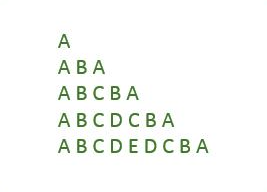# C Program to Print Palindrome Triangle Pattern

Here is a C program to print palindrome triangle pattern of n rows. Palindrome triangle pattern of 5 rows:#### Required Knowledge

Algorithm to print triangle pattern of palindrome strings
This program is silimar to right triangle star pattern program.
• Take the number of rows(N) of right triangle as input from user using scanf function.
• Number of characters in Kth row is always 2K+1. 0th row contains 1 character, 1st row contains 3 characters, 2nd row contains 5 charcaters and so on.
• In any row, we will first print the characters in increasing order of their ASCII value till mid of the row and then in decreasing order.
• We will use two for loops to print right triangle of palindrome strings.
• Outer for loop will iterate N time(from i = 0 to 2N-2 in increment of 2). Each iteration of outer loop will print one row of the pattern.
• Each iteration of inner loop will print a palindrome string as explained above.

Here is the matrix representation of the above mentioned pattern. The row numbers are represented by i whereas column numbers are represented by j.## C program to print palindrome triangle pattern

```#include<stdio.h>

int main() {
int i, j, rows, count=0;

printf("Enter the number of rows\n");
scanf("%d", &rows);

for (i = 0; i < 2*rows; i=i+2) {
for (j = 0; j <= i; j++) {
printf("%c", 'A'+count);
if(j < i/2)
count++;
else
count--;
}
count = 0;
printf("\n");
}
return(0);
}
```
Output
```Enter the number of rows
5
A
ABA
ABCBA
ABCDCBA
ABCDEDCBA
```

Related Topics
 C program prime number triangle pattern C program binary triangle pattern C program same row element triangle pattern C program hollow pyramid star pattern C program binary rectangle pattern C program hollow diamond star pattern C program heart shape star pattern C program hollow square star pattern C program rectangle star pattern List of all C pattern programs